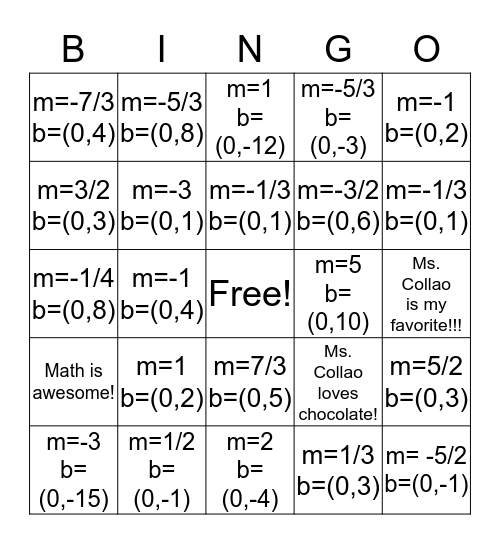# Finding slope and y-int from EquationThis bingo card has a free space and 26 words: m=7/3 b=(0,5), m=1/3 b=(0,3), m=-1/3 b=(0,1), m=1/2 b=(0,-1), m=-1 b=(0,2), m=-7/3 b=(0,4), m=3/2 b=(0,3), m=-5/3 b=(0,8), Ms. Collao is my favorite!!!, Ms. Collao loves chocolate!, m=-3/2 b=(0,6), m=-1/4 b=(0,8), m=5/2 b=(0,3), m=-3 b=(0,-15), m=-1 b=(0,4), m=-3 b=(0,-3), m=-5/3 b=(0,-3), Math is awesome!, m=1 b=(0,-12), m= -5/2 b=(0,-1), m=-1/3 b=(0,1), m=2 b=(0,-4), m=5 b=(0,10), m=1 b=(0,2), m=-3 b=(0,1) and m=2 b=(0,-5).

⚠ This card has duplicate items: m=-1/3 b=(0,1) (2)

## Play Online